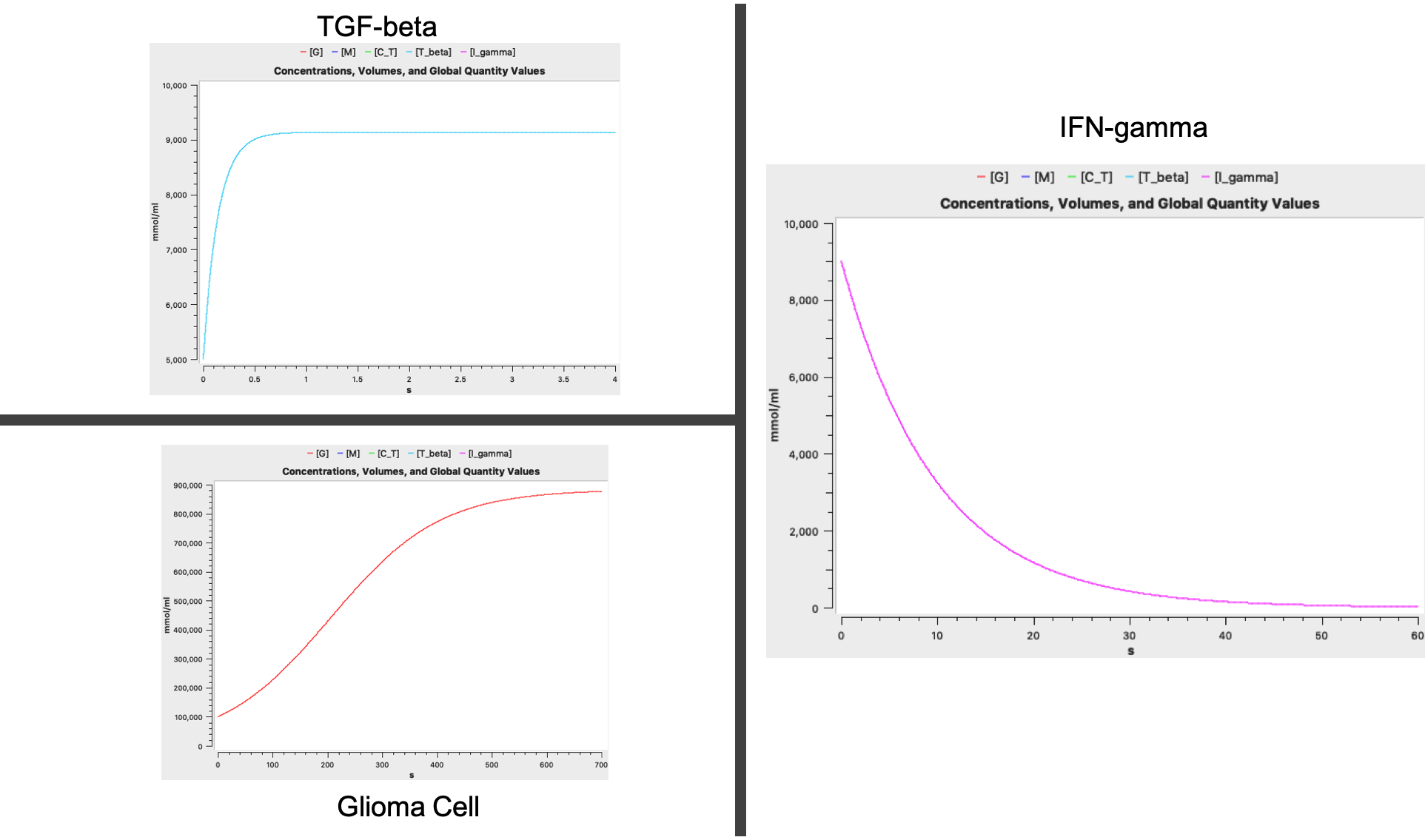## Khajanchi2017 - Uniform Persistence and Global Stability for a Brain Tumor and Immune System InteractionModel Identifier
BIOMD0000000921
Short description
This paper describes the synergistic interaction between the growth of malignant gliomas and the immune system interactions using a system of coupled ordinary di®erential equations (ODEs). The proposed mathematical model comprises the interaction of glioma cells, macrophages, activated Cytotoxic T-Lymphocytes (CTLs), the immunosuppressive factor TGF- and the immuno-stimulatory factor IFN-. The dynamical behavior of the proposed system both analytically and numerically is investigated from the point of view of stability. By constructing Lyapunov functions, the global behavior of the glioma-free and the interior equilibrium point have been analyzed under some assumptions. Finally, we perform numerical simulations in order to illustrate our analytical ¯ndings by varying the system parameters.
Format
SBML (L2V4)
Related Publication
• Uniform Persistence and Global Stability for a Brain Tumor and Immune System Interaction• Subhas Khajanchi
• Biophysical Reviews and Letters , 11/ 2017 , Volume 12 , Issue 4 , pages: 1-22 , DOI: 10.1142/S1793048017500114
• Department of Mathematics, Bankura University Bankura 722155, West Bengal, India subhaskhajanchi@gmail.com
• This paper describes the synergistic interaction between the growth of malignant gliomas and the immune system interactions using a system of coupled ordinary di®erential equations (ODEs). The proposed mathematical model comprises the interaction of glioma cells, macrophages, activated Cytotoxic T-Lymphocytes (CTLs), the immunosuppressive factor TGF- and the immuno-stimulatory factor IFN-. The dynamical behavior of the proposed system both analytically and numerically is investigated from the point of view of stability. By constructing Lyapunov functions, the global behavior of the glioma-free and the interior equilibrium point have been analyzed under some assumptions. Finally, we perform numerical simulations in order to illustrate our analytical ¯ndings by varying the system parameters.
Contributors
Submitter of the first revision: Mohammad Umer Sharif Shohan
Submitter of this revision: Mohammad Umer Sharif Shohan

is (2 statements)
BioModels Database BIOMD0000000921
BioModels Database MODEL2003110001

hasTaxon (1 statement)
Taxonomy Homo sapiens

hasProperty (1 statement)
Mathematical Modelling Ontology Ordinary differential equation model

isDerivedFrom (2 statements)
Mathematical Modelling Ontology Ordinary differential equation model
Taxonomy Homo sapiens

isDescribedBy (1 statement)

Curation status
Curated

Modelling approach(es)

Tags

#### Connected external resources

SBGN view in Newt Editor

Name Description Size Actions

### Model files

khajanchi2017.xml SBML L2V4 representation of Khajanchi2017 - Uniform Persistence and Global Stability for a Brain Tumor and Immune System Interaction 74.87 KB Preview | Download

khajanchi2017.cps COPASI version 4.27 (Build 197) representation of Khajanchi2017 - Uniform Persistence and Global Stability for a Brain Tumor and Immune System Interaction 111.58 KB Preview | Download
khajanchi2017.sedml SEDML L2V1 Khajanchi2017 - Uniform Persistence and Global Stability for a Brain Tumor and Immune System Interaction 3.73 KB Preview | Download
• Model originally submitted by : Mohammad Umer Sharif Shohan
• Submitted: Mar 11, 2020 4:10:22 PM
##### Revisions
Legends
: Variable used inside SBML models

Species
Species Initial Concentration/Amount
C T

C12543
1.0 mmol
G

glioma cell
100000.0 mmol
M

macrophage
100000.0 mmol
I gamma

Interferon Gamma
9000.0 mmol
T beta

C30098
5000.0 mmol
Reactions
Reactions Rate Parameters
C_T => compartment*mu1*C_T mu1 = 0.007
C_T => ; G compartment*alpha4*G/(G+k3)*C_T alpha4 = 0.1694; k3 = 334450.0
G => ; T_beta, M, C_T compartment*1/(T_beta+e1)*(alpha1*M+alpha2*C_T)*G/(G+k1) alpha2 = 0.12; k1 = 27000.0; alpha1 = 1.5; e1 = 10000.0
=> M compartment*r2*M*(1-M/M_max) M_max = 1.0; r2 = 0.3307
I_gamma => compartment*mu3*I_gamma mu3 = 0.102
=> T_beta compartment*s1 s1 = 63305.0
=> T_beta; G compartment*b1*G b1 = 5.75E-6
=> G compartment*r1*G*(1-G/G_max) r1 = 0.01; G_max = 882650.0
M => ; G compartment*alpha3*G/(G+k2)*M alpha3 = 0.0194; k2 = 27000.0
=> C_T; G, T_beta compartment*a2*G/(k5+T_beta) k5 = 2000.0; a2 = 0.0Curator's comment:
(added: 11 Mar 2020, 16:09:12, updated: 11 Mar 2020, 16:09:12)
The model was encoded in COPASI 4.27 (Build 197) and the figure was reproduced using COPASI. Several Parameters were missing. The initial concentration was set to G = 10000, M = 10000, C_T = 1, TGF-beta = 5000, INF-gamma = 9000 The parameter M_max was missing which was set to 1